﻿ Sobolev方程的一个紧致差分格式 A Compact Finite Difference Scheme for Sobolev Equations

Pure Mathematics
Vol.07 No.01(2017), Article ID:19461,9 pages
10.12677/PM.2017.71001

A Compact Finite Difference Scheme for Sobolev Equations

Xin Jing*, Luming Zhang

School of Mathematics, Nanjing University of Aeronautics and Astronautics, Nanjing Jiangsu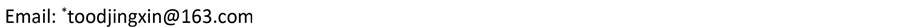Received: Dec. 17th, 2016; accepted: Jan. 2nd, 2017; published: Jan. 5th, 2017ABSTRACT

A compact finite difference scheme is presented for Sobolev equations. It is proved by the discrete energy method that the compact scheme is unconditionally stable and convergent in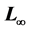norm, and the order of convergence is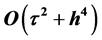. The numerical experiment results show that the theory is accurate.

Keywords:Sobolev Equations, Compact Finite Difference Scheme, Convergence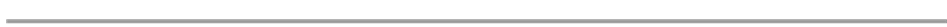Sobolev方程的一个紧致差分格式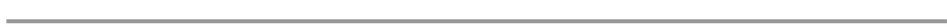1. 引言(1)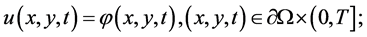(2)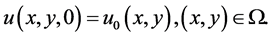(3)

Sobolev方程是一个重要的数学物理方程，被用来描述流体穿过岩石或土壤及不同介质中的流体运动，土壤中湿气的迁移等    。由于实际问题中Sobolev方程的源函数、初边值或计算域会很复杂，故有效的求解方法是求其数值解。

2. 差分格式的建立

2.1. 一些记号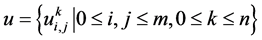上的网格函数，记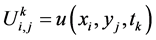，类似地定义网格函数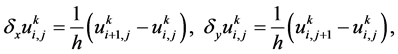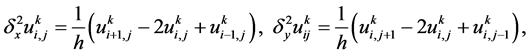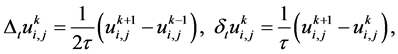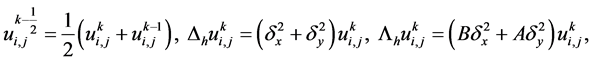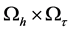上的网格函数，定义范数：2.2. 差分格式(4)(5)(6)(7)(8)(9)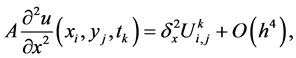(10)(11)(12)(13)(14)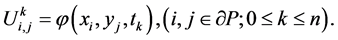(15)(16)(17)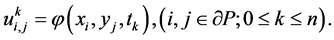(18)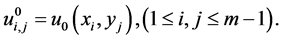(19)

3. 数值解的先验估计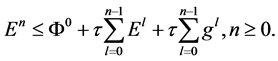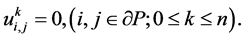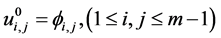(20)(21)(22)(23)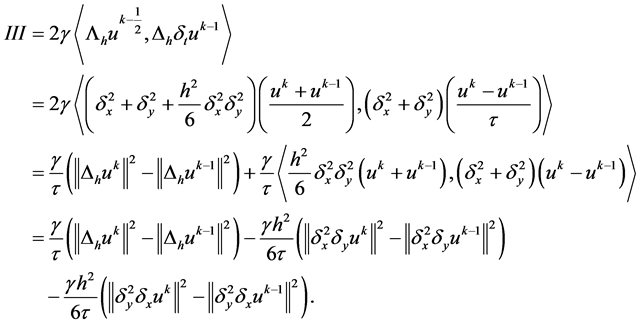(24)(25)(26)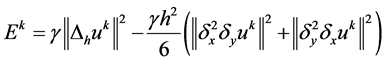。则(26)式有：(27)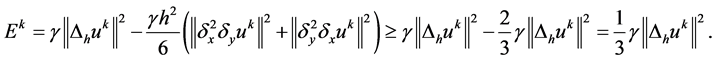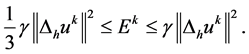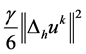移项，不等式两边再同乘以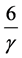，有：4. 差分格式的收敛性与稳定性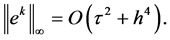(28)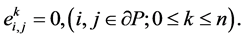(29)(30)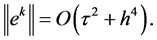5. 数值算例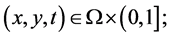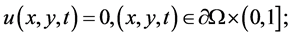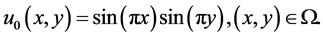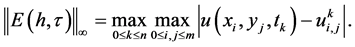Table 1. Errors of numerical solution and convergence rate of example at different step sizes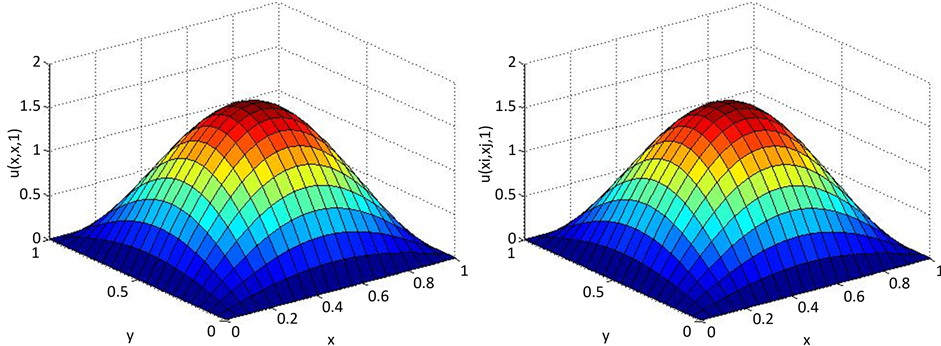Figure 1. The surface of exact solution and numerical solution when t = 1 (h = 1/20, τ = 1/400)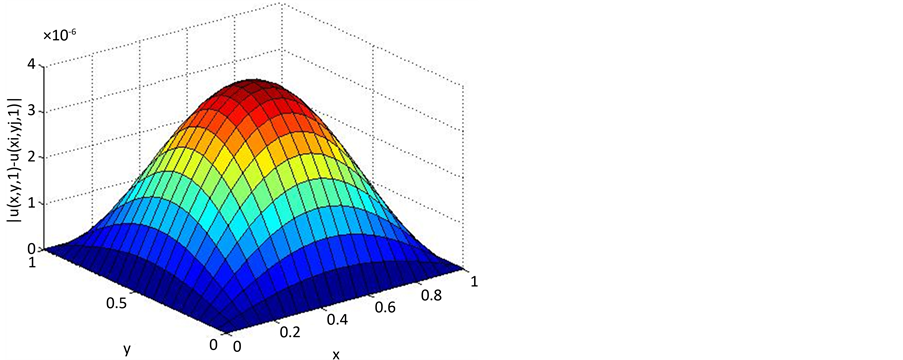Figure 2. The surface of absolute error when t = 1 (h = 1/20, τ = 1/400)

A Compact Finite Difference Scheme for Sobolev Equations[J]. 理论数学, 2017, 07(01): 1-9. http://dx.doi.org/10.12677/PM.2017.71001

1. 1. Barenblett, G.I., Zheltov, I.P. and Kochian, I.N. (1990) Basic Concepts in the Theory of Homogeneous Liquids in Fissured Rocks. Journal of Applied Mathematics and Mechanics, 24, 1286-1303. https://doi.org/10.1016/0021-8928(60)90107-6

2. 2. 施德明. 非线性湿气迁移方程的边值问题[J]. 应用数学学报, 1990, 13(1): 33-40.

3. 3. Ting, T.W. (1974) A Cooling Process According to Two-Temperature Theory of Heat Conduction. Journal of Mathematical Analysis and Applications, 45, 23-31. https://doi.org/10.1016/0022-247X(74)90116-4

4. 4. 郭会, 芮洪兴. Sobolev方程的最小二乘Galerkin有限元方法[J]. 应用数学学报, 2006, 29(4): 610-618.

5. 5. 郭玲, 陈焕贞. Sobolev方程的H1-Galerkin混合有限元方法[J]. 系统科学与数学, 2006, 26(3): 302-314.

6. 6. 何斯日古楞, 李宏. Sobolev方程的时间间断Galerkin有限元方法[J]. 高校应用数学学报, 2011, 26(4): 467-473.

7. 7. 李宏, 罗振东, 安静, 孙萍. Sobolev方程的全离散有限体积元格式及数值模拟[J]. 计算数学, 2012, 34(2): 164- 172.

8. 8. 李宏, 周文文, 方志朝. Sobolev方程的CN全离散化有限元格式[J]. 计算数学, 2013, 35(1): 41-47.

9. 9. 史艳华, 石东洋. Sobolev方程新混合元方法的高精度分析[J]. 系统科学与数学, 2014, 34(4): 452-463.

10. 10. Zhao, Z.H., Li, H. and Luo, Z.D. (2016) A New Space-Time Continuous Galerkin Method with Mesh Modification for Sobolev Equations. Journal of Mathematical Analysis and Applications, 400, 86-105. https://doi.org/10.1016/j.jmaa.2016.03.035

11. 11. 罗振东, 张博. Sobolev方程基于POD的降阶外推差分算法[J]. 应用数学和力学, 2016, 37(1): 108-116.

12. 12. Liao, H.L. and Sun, Z.Z. (2010) Maximum Norm Error Bounds of ADI and Compact ADI Methods for Solving Parabolic Equations. Numerical Methods Partial Differential Equations, 26, 37-60. https://doi.org/10.1002/num.20414